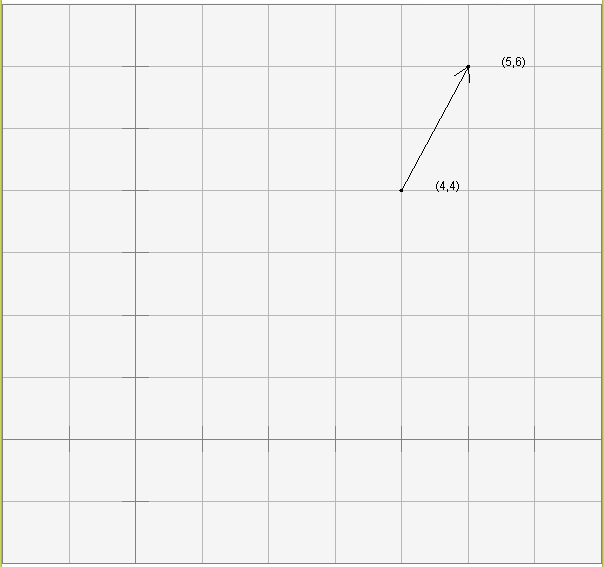Your picture should look like the one below.

# TranslationSometimes the operation of adding a vector to a point is called translation. The original point is sometimes said to have been "translated to a new location."

The vocabulary here might be confusing. In mathematical terms, the sum of a vector and a point yields a new point. The first point remains unchanged. But in computer graphics terms it is nice to think about pictures "moving across the screen" when displacement vectors are added to their points. So sometimes people talk about "moving a point" and talk about the "old point" and the "new point".

### QUESTION 21:

We have seen:

• vector + vector
• point + vector
• point - point

Do you suppose that this operation can be done:

• point + point